# 14-240/Tutorial-Sep30

## Boris

#### Problem

Find a set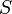$S$ of two elements that satisfies the following:

(1)$S$ satisfies all the properties of the field except distributivity.

(2)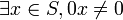$\exists x \in S, 0x \neq 0$.

Solution:

Let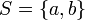$S = \{ a, b \}$ where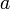$a$ is the additive identity and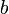$b$ is the multiplicative identity and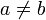$a \neq b$. After trial and error, we have the following addition and multiplication tables: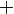$+$$a$$b$$a$$a$$b$$b$$b$$a$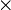$\times$$b$$a$$b$$b$$a$$a$$a$$b$

We verify that$S$ satisfies (1). By the addition and multiplication tables, then$S$ satisfies closure, commutativity, associativity and existence of identities and inverses. Since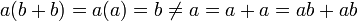$a(b + b) = a(a) = b \neq a = a + a = ab + ab$, then$S$ does not satisfy distributivity. Then$S$ satisfies (1).

We verify that$S$ satisfies (2). Since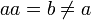$aa = b \neq a$, then$S$ satisfies (2).

#### Elementary Errors in Homework

(1) Prove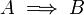$A \implies B$. Assume$A$ and derive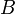$B$. It is not the other way around.

(2) Prove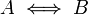$A \iff B$. Show that$A \implies B$ and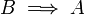$B \implies A$.

(3) This is for Boris's section only. When a proof requires a previous result, there are two possibilities:

(a) The result is already proved in class or in a previous homework. Then state the result and use it without proof.
(b) The result is neither proved in class nor in a previous homework. Then reference it in the textbook or prove it yourself.